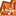cancel
Showing results for
Did you mean:

## Nested struct type not supported pyspark errorNew Contributor III

I am attempting to apply a function to a pyspark DataFrame and save the API response to a new column and then parse using `json_normalize`. This works fine in pandas, however, I run into an exception with `pyspark`.

`````` import pyspark.pandas as ps

import pandas as pd

import requests

def get_vals(row):

# make api call

return row['A'] * row['B']

# Create a pandas DataFrame

pdf = pd.DataFrame({'A': [1, 2, 3], 'B': [4, 5, 6]})

# apply function - get api responses

pdf['api_response'] = pdf.apply(lambda row: get_vals(row), axis=1)

pdf.sample(5)

# Unpack JSON API Response

try:

dff = pd.json_normalize(pdf['api_response'].str['location'])

except TypeError as e:

print(f"Error: {e}")

print(f"Problematic data: {data['data']}")

# To pySpark DataFrame

psdf = ps.DataFrame(df)

Expected output is a `json` normalized DataFrame. When I attempt to apply the function over a `pyspark` DataFrame, it throws an exception:

``````psdf['api_response'] = psdf.apply(lambda row: get_vals(row), axis=1)

---------------------------------------------------------------------------

TypeError                 Traceback (most recent call last)

File <command-4372401754138893>:2

1

----> 2 psdf['api_response'] = psdf.apply(lambda row: get_vals(row), axis=1)

TypeError: Nested StructType not supported in conversion from Arrow: struct<data: struct<geographies: ``````

2 REPLIES 2Anonymous
Not applicable

@Keval Shah​ :

The error message suggests that the issue is with the schema of the psdf DataFrame, specifically with the nested struct type of the api_response column.

Unfortunately, PySpark does not support nested struct types when converting from Arrow format, which is used internally by PySpark DataFrames. As a result, you cannot directly apply a function to a PySpark DataFrame that returns a nested struct type.

One workaround is to use the pandas_udf function in PySpark, which allows you to apply a Pandas UDF (user-defined function) to a PySpark DataFrame. You can define a Pandas UDF that takes a Pandas DataFrame as input, applies the function to the DataFrame, and returns a new Pandas DataFrame with the normalized JSON data. Here's an example of how you can modify your code to use pandas_udf:

``````import pyspark.sql.functions as F
from pyspark.sql.types import StructType, StructField, DoubleType

# Define the schema of the output DataFrame
output_schema = StructType([
StructField("location.latitude", DoubleType()),
StructField("location.longitude", DoubleType())
])

# Define the function that applies the API call and normalizes the JSON response
def api_call_udf(pdf):
# Apply the API call to the input Pandas DataFrame
pdf['api_response'] = pdf.apply(lambda row: get_vals(row), axis=1)
# Normalize the JSON data using pandas.json_normalize
normalized_df = pd.json_normalize(pdf['api_response'].str['location'])
# Return the normalized Pandas DataFrame as an Arrow table
return normalized_df.to_arrow()

# Convert the PySpark DataFrame to a Pandas DataFrame
pdf = psdf.to_pandas()

# Apply the function using pandas_udf
normalized_df = psdf.withColumn("output", F.pandas_udf(api_call_udf, output_schema)(F.struct([F.col(x) for x in pdf.columns])))

# Convert the output PySpark DataFrame back to a PySpark Pandas DataFrame
psdf_output = ps.DataFrame(normalized_df)``````

In this code, we define a schema for the output DataFrame and define a function that applies the API call to the input Pandas DataFrame, normalizes the JSON data using pandas.json_normalize, and returns the normalized Pandas DataFrame as an Arrow table. We then convert the PySpark DataFrame to a Pandas DataFrame, apply the function using pandas_udf, and convert the output PySpark DataFrame back to a PySpark Pandas DataFrame.Anonymous
Not applicable

Hi @Keval Shah​

Thank you for posting your question in our community! We are happy to assist you.

To help us provide you with the most accurate information, could you please take a moment to review the responses and select the one that best answers your question?

This will also help other community members who may have similar questions in the future. Thank you for your participation and let us know if you need any further assistance!Welcome to Databricks Community: Lets learn, network and celebrate together

Join our fast-growing data practitioner and expert community of 80K+ members, ready to discover, help and collaborate together while making meaningful connections.